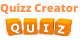Create Quiz

# Beer–Lambert law Quiz## Beer–Lambert law Quiz Test, Are you a Science student and loves to get more knowledge of it from every corner. The Beer-Lambert law or Beer's law is the linear relationship between absorbance and concentration of an absorbing species. Johann Heinrich Lambert studied the findings of Pierre Bougruer. The law included path length as a variable that affect absorbance. Later Beer modified the law by addition of concentration of solutions. The absorbance is directly proportional to the concentration of the solution and to the length of the light path. The constant ε in this law is called molar absorptivity or molar extinction coefficient and is a measure of the probability of the electronic transition. The larger the molar absorptivity, the more probable the electronic transition. The beer- Lambert Law has its own limitations. At high concentration, the value measurement can be inappropriate or incorrect. This happens due to strong intermolecular forces between the molecules due to which there is very less space between the molecules. The high concentration also change the molar absorptivity and refractive index of the solution, thus deviating the linear path between absorbance and concentration. This law has been founded incomplete because this law does not say about anything of the nature of light and it does not consider the angle of incident beam and properties of the material on which light is concentrating.For each wavelength of light passing through the spectrometer, the intensity of the light passing through the reference cell is measured. The intensity of the light passing through the sample cell is also measured for that wavelength. An absorbance of 0 at particular wavelength means that no light of that wavelength is absorbed. In most of the diagram, the absorbance ranges from 0 to 1. To get more knowledge about it, let’s get back to quiz.

You can mute/unmute sounds from here

200-300
340-850
380-750
500-900

## When plotting absorbance against concentration of a colored solution, which obey's Beer's Law, what procedure would be followed to obtain a straight line graph with a positive slop

Plot on semi-logarithmic graph paper with absorbance on semi-log axis
Plot on arithmetic graph paper
Plot on log-log paper
Convert to %T and plot on arithmetic graph paper
Plot on semi-logarithmic graph paper with concentration on semi-log axis

## According to the Beer-Lambert Law, absorbance is:

Inversely proportional to the concentration
Directly proportional to the concentration
Directly proportional to the transmittance
Directly proportional to the log of the concentration

## In measuring the absorbance of a compound at 250 nanometers on a spectrophotometer, we have to use:

An infra-red sensitive photocell
A tungsten lamp
A hydrogen discharge lamp
A mercury discharge lamp

## The intensity of scattered light from suspended particles?

Holds true in dilute solutions and small particle size
Holds true as particle size increases
Directly proportional to wavelength
All of the above

0.80
0.40
0.20
0.10

0.80
0.40
0.20
0.10

200 nm
300 nm
400 nm
500 nm

## A component of a spectrophotometer that isolates radiant energy of a specific wavelength and excludes that of other wavelengths is called a(n):

Fluorometer
Incandescent lamp
Monochromator
Photomultiplier tube

## To determine if the wavelength selector of a broad-bandpass spectrophotometer is actually isolating the desired wavelength, a __________ should be performed.

Wavelength calibration with didymium filter
Linearity check with standard solutions
Spectral scan of a chromogen